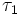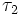# Coarser topology

(Redirected from Coarsest topology)
Jump to: navigation, search
This article is about a basic definition in topology.
VIEW: Definitions built on this | Facts about this | Survey articles about this
View a complete list of basic definitions in topology

## Definition

### Symbol-free definition

Given two topologies on a set, one is said to be coarser than the other if the following equivalent conditions are satisfied:

• Every set that is open as per the first topology, is also open as per the second
• Every set that is closed as per the first topology, is also closed as per the second
• The identity map is a continuous map from the second topology to the first

### Definition with symbols

Let$X$ be a set and$\tau_1$ and$\tau_2$ be two topologies on$X$. We say that$\tau_1$ is coarser than$\tau_2$ if the following equivalent conditions are satisfied:

• Any open set for$\tau_1$ is also open for$\tau_2$
• Any closed set for$\tau_1$ is also closed for$\tau_2$
• The identity map$(X,\tau_2) \to (X,\tau_1)$ is a continuous map

The opposite notion is that of finer topology. In this case,$\tau_2$ is finer than$\tau_1$.

## Related notions

### Universal constructions

The trivial topology (the topology where the only open subsets are the whole space and the empty set) is the coarsest possible topology on a set. We are often interested in the coarsest possible topology on a set subject to additional conditions. For instance, the subspace topology is the coarsest topology on a subset to make the inclusion map continuous. More generally, pullbacks are given the coarsest possible topology to make the maps from them continuous.

### Effect on topological space properties

Moving from a particular topology on a set to a coarser topology might have various kinds of effect on topological space properties. A list of topological space properties that are preserved on passing to coarser topologies is available at: